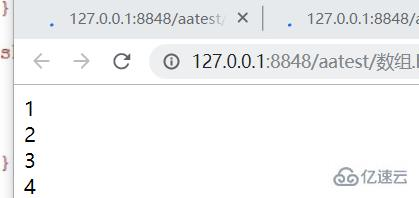# javascript中如何调用函数

javascript中调用函数的方法：1、使用“对象.函数名()”语句调用；2、使用“函数名().call(调用者,参数1 ,参数2 , .....);”语句调用；3、使用“函数名().apply(调用者,参数数组);”语句调用。

### 函数的三种实现方式

```		<script type="text/javascript">
function show(name){
document.write(name+"  hellow")
}
show('laoli');
</script>```

```		<script type="text/javascript">

var f=function(name){
document.write('name+"  hellow")
}
f('laoli');
</script>```

• Function中f大写

• 参数列表和函数执行体要加引号

• 以分号结束

```		<script type="text/javascript">
var f = new Function('name', 'alert(name+"你好");');
f('laoli');
</script>```

```		<script type="text/javascript">
function show(){
document.write('我是命名函数')
}
var f=show();
f();//函数调用
</script>```

### 函数中变量与函数访问

```		<script type="text/javascript">
var num='laoli';
var f=function(num){
document.write(num+'真可爱');

function show(){
document.write('他不是女人')
}
show();//调用show(）函数
}
//执行函数
f(num);
</script>```

### 函数调用三种方式

• 对象.函数应用

• call方法调用函数 函数应用.( 调用者,参数1 ,参数2 , .....)

• apply方法调用函数  apply(调用者,参数数组)

```		<script type="text/javascript">
//创建命名函数
function show(name, age) {
document.write(name + '是男人，他' + age + '岁');
}
//对象.函数应用
window.show('小明', '30');
//all方法调用函数 函数应用.( 调用者,参数1 ,参数2 , .....)
show.call(window, '小明', '30');
//apply方法调用函数  apply(调用者,参数数组)
show.apply(window, ['小明', '30']);
</script>```

```		<script type="text/javascript">
//show传入两个参数 1个数组 1个函数
function show(arr, func) {
//func.call(window, arr);
func.apply(window, [arr])
}
show([1, 2, 3, 4], function(arr) {
for (i in arr) {
document.write(arr[i] + '<br/>')
}
});
</script>```